# z-mR Chart Helpz-mR charts are used to monitor short production runs. This allows you to plot different products on the same chart. The “z” values for each product are plotted on the z chart. z is defined as the following:

• z = (x – Nominal)/s

where:

• x = sample results
• Nominal = nominal value for the product
• s= standard deviation for the product

You may use the product average in place of the nominal value. The moving range chart plots the range between consecutive z values.

### Data Entry

The data are entered into a worksheet as shown below (using the first part of the data in the example workbook). The data does not have to start in A1. It can be anywhere on the worksheet. The data can be in rows or columns. This example has the data in columns since the sample identifiers are in a single column. In the example below, the sample numbers are in one column, the product identifiers are in one column and the sample results (values) are in one column. With the z-mR chart, you have the option of running the chart using the nominal for the product or the average for the product. If you use the nominal, the nominal values must be entered in one column. If you select the average option, the software calculates the average to use for each product. With the average option, you do not need the column with the nominal values.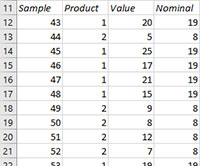### Creating a New z-mR

1. Select the data on the worksheet to be included in the analysis.

2. Select “Variable” from the “Control Charts” panel on the SPC for Excel ribbon.

3. The input screen for variable control charts is displayed. The z-mR is listed on the second tab (Individuals Charts) of input screen.

Select it and OK

4. The input screen for the z-mR Chart will show. The software assumes that the data are entered as above. You can edit the ranges if needed here. Enter a name for the chart. This is all that is needed to make the z-mR chart. In this example, the name “Short Run” is used as the name of the z-mR chart. This name must be unique – no other worksheet tabs can have this name.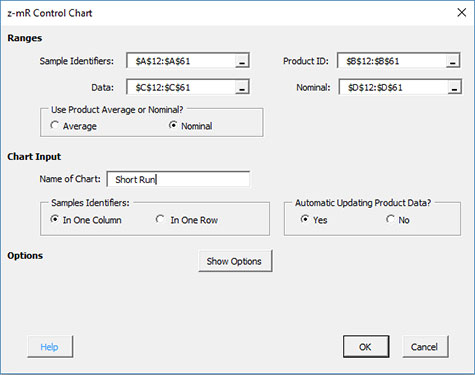• Sample Identifiers: worksheet range containing the sample identifiers
• Product ID: worksheet range containing the product identifiers
• Data: worksheet range containing the sample result
• Nominal: worksheet range containing the nominal values (not required if the product average option is selected)
• Use Product Average or Nominal?
• Average: select this option to use each product’s average in the calculations of z
• Nominal: select this option to use each product’s nominal value in the calculation of z
• Name of Chart: chart name – must be a unique name in the workbook and is limited to 25 characters
• Samples: this information is used to search for new data when updating the chart (see below)
• In 1 column: the sample identifiers are in one column
• In 1 row: the sample identifiers are in one row
• Automatic Updating of Limits: option controls whether the product metrics are updated as new data are added, default value is Yes; set this to No to stop the product metrics from updating
• Show Options: selecting this button shows the various options for this chart (see options below)
• Out of Control Tests
• Titles and Formats
• Enter Product Statistics
• Box-Cox Transformation
• Chart Location
• Select OK to create the z-mR chart
• Select Cancel to exit the software

5. Once you have entered the information, select OK and the z-mR chart will be generated. An example based on the data above is shown below.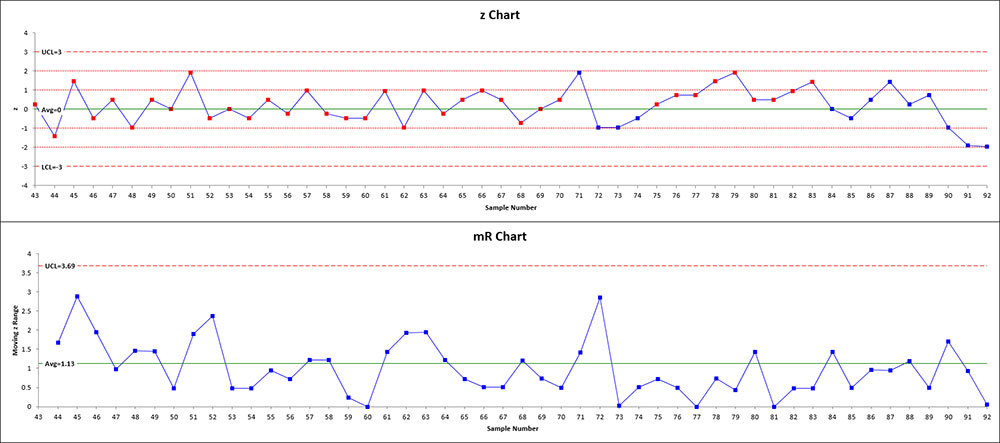6. The software also generates a report with the metrics for each product. This report is placed on the worksheet called “z Chart Info”. This gives the metrics used in the calculations. The results from this chart are shown below. The information lists the date and time the chart was made, the name of the chart, the average and sigma for each product as well as the Box-Cox lambda value if that transformation option was selected (see below).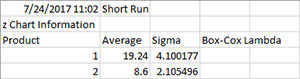### Options for the z-mR chart

On the input screen for the z-mR chart, there is a button labeled “Show Options”. If you select that button, the input screen will show the options available for the chart. It is not required to select any of these options.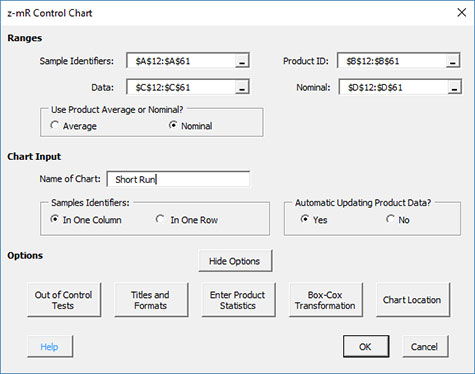The options are explained below.

Out of Control Tests

If you select the Out of Control Tests, the input screen, Tests for Out of Control Points, is displayed below.These options include:

• Point beyond the control limits: out of control if a point that is above the upper control or below the lower control
• k points in a row trending up or down: out of control if there are k points in a row trending upward or trending downward; two points in a row that are equal break this trend
• k out of k+1 points in Zone A or beyond: Zone A is two standard deviations of what is being plotted above the average and below the average
• k out k+1 points in Zone B or beyond: Zone B is one standard deviation of what is being plotted above the average and below the average
• k points in a row in Zone C or beyond (one side of avg): Zone C is the zone between the average and one standard deviation above the average on the top half of the chart and the average and one standard deviation below the average on the bottom half of the chart; out of control of there is a run of k points in a row above or below the average
• Mixture Test: k points in a row with none in Zone C: out of control if there are k points in a row not in Zone C on either side of the average
• Stratification Test: k points in a row within Zone C: out of control if there are k points in a row in Zone C on either side of the average
• Overcontrol: k points in row alternating up and down: out of control if k points in a row are such that the values alternate up and down
• Set Selected Tests as Defaults: this will set the selected test and k values as defaults for the type of chart
• Reset Tests to Program Defaults: resets the tests to the original program defaults for the type of chart.
• Plot 1 and 2 Sigma Lines (for zone tests): select this option to plot the 1 and 2 sigma lines on on the control chart; this option is overridden by the option to add two additional lines on the Control Limits Options.

Note: only four tests apply to the mR chart:

• Point beyond the control limits
• k points in a row trending up or down
• k points in a row in Zone C or beyond (one side of avg)
• Overcontrol: k points in row alternating up and down: out of control if k points in a row are such that the values alternate up and down

Titles and Formats

If you select the “Title and Formats” button, the following screen is displayed: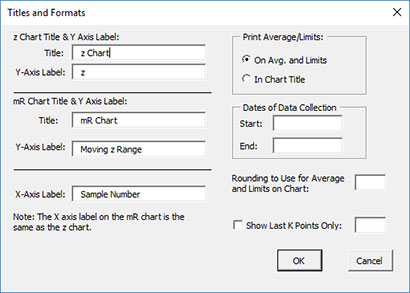The options include:

• z Chart Title & Y Axis Label:
• Title: title at the top of the chart; default is “z Chart”
• Y-Axis Label: label to put on the chart vertical axis; default is “z”
• mR Chart Title & Y Axis Label:
• Title: title at the top of the range chart; default is “mR Chart”
• Y-Axis Label: label to put on the range chart vertical axis; default is “Moving z Range”
• X-Axis Label: label for the horizontal axis for both the z chart and the mR chart; default is “Sample Number”
• Print Average/Limits:
• On Avg. and Limits: prints the values of the average and control limits on the average and control limit lines on the control chart (this is the default)
• In Chart Title: prints the values of the average and control limits in the control chart title
• Dates of Data Collection: enter the start and end dates of data collection; optional; dates will be plotted at the bottom of the chart
• Rounding to Use for Average and Limits on Chart: used to control the number of decimal places in the values of the average and control limits printed on the chart; default is estimated by the software
• Show Last K Points Only: option to show only the last K points of the chart; calculations include all the data but only the last K points will be shown
• Select OK to use selected options
• Select Cancel to revert to previous options

Enter Product Statistics Option

You have the option to enter the average and sigma metrics for each product. If entered, these will be used in place of the calculated values. To use this option, you must enter the product metrics in the worksheet before you make the z-MR chart. The data entry must in the following form: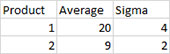When the z-mR chart input screen is shown, select “Show Options” and then select “Enter Product Statistics”. The input screen below is shown.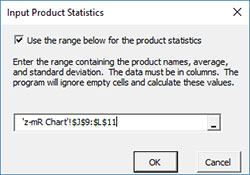Select the “Use the range below for the product statistics” and then select the range on the worksheet containing the information. Select OK. The program will use the entered values in place of the calculated metrics.

Box-Cox Transformation

Selecting the “Box-Cox Transformation” button displays the following screen: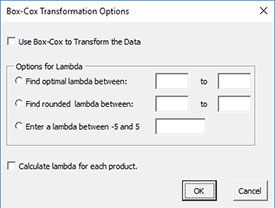The options are:

• Use Box-Cox to Transform the Data: selecting this option reveals the bottom part of the input form
• Options for Lambda
• Find optimal lambda between: finds the value of lambda that minimizes the standard deviation between the default values of -5 and 5
• Find rounded lambda between: finds the rounded value of lambda that minimizes the standard deviation between the default values of -5 and 5; rounded value of lambda are -5, -4, -3, -2, -1, -.5, 0, .5, 1, 2, 3, 4 and 5
• Enter a lambda between -5 and 5: enter a value of lambda to use between the values of -5 and 5
• Calculate lambda for each split limit: this option will calculate the value of lambda for each set of split control limits
• Select OK to use selected options
• Select Cancel to revert to previous options

Note:

• The default values of -5 and 5 can be changes when finding the optimal or rounded values of lambda, but must stay between -5 and 5.
• The standard deviation that is minimized is based on the option you selected to estimate sigma for the control chart (see Control Limit Options)
• A separate Box-Cox transformation is done for each product

Chart Location

If you select the “Chart Location” button, the following screen is displayed.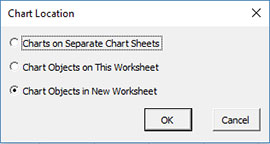The options are:

• Charts on Separate Chart Sheets: places the z chart on one chart sheet and the mR chart on another chart sheet
• Chart Objects on This Worksheet: places both the z and mR chart on the worksheet containing the data
• Chart Objects in a New Worksheet: places both the z and mR chart on a new worksheet
• Select OK to use selected options
• Select Cancel to revert to previous options

Notes:

• Once a control chart is made, you can move the chart to new locations in the workbook. The software will find the chart when updating.
• This option is not available when a chart’s options are updated since you can manually move the charts to new locations in the workbook

### Updating the z-mR Chart with New Data

The z-mR chart can be easily updated with new data after it has been added to the spreadsheet. Please see Updating Control Charts with New Data to see how the software finds the new data and updates the chart.

### Changing the Options for the z-mR Chart

You can change the current options for a z-mR chart (e.g., adding a Box-Cox transformation) by selecting “Options” on the Updating/Options panel on the SPC for Excel Ribbon.

The list of available charts in the workbook will be displayed. Select the chart you want to change options for. The input screen for that chart will be shown and changes can be made. See Changing Chart Options for more information

In addition, once a control chart is made, there are numerous actions you can take on the chart including splitting control limits, removing points from the calculations, adding comments, selecting the range on which to base control limits, etc. Please see Control Chart Actions for details.